﻿ 机器学习在湍流模型构建中的应用进展文章快速检索 高级检索
 空气动力学学报2019, Vol. 37Issue (3): 444-454  DOI: 10.7638/kqdlxxb-2019.0036### 引用本文ZHANG W W, ZHU L Y, LIU Y L, et al. Progresses in the application of machine learning in turbulence modeling[J]. Acta Aerodynamica Sinica, 2019, 37(3): 444-454.### 文章历史

Progresses in the application of machine learning in turbulence modeling
ZHANG Weiwei, ZHU Linyang , LIU Yilang , KOU Jiaqing
Northwestern University of Technology Aviation School, Xi'an 710072, China
Abstract: With the development of high performance computer and data sharing platform, a large number of high fidelity turbulence data can be obtained. Recently, due to the evolution of artificial intelligence, like deep neural network, data-driven machine learning methods have been adopted to quantify the model uncertainty and improve and construct turbulence models. The combination of big turbulence data and artificial intelligence becomes a new area of turbulence research. Although some encouraging results have been achieved, there are still many difficulties and challenges, such as the generalization ability and robustness of the models, etc. The modeling process involves various aspects including data process, feature selection and selection and optimization of the model framework, etc. This paper analyzes and summarizes the main research progress from two aspects:the implementation methods of machine learning in turbulence modeling and the different model targets. Besides, the challenges and future works in this area are also discussed.
Keywords: turbulence    machine learning    artificial intelligence    deep neural networks    data-driven
0 引言

RANS模型大多基于涡黏假设，在附着流中容易取得较好的计算结果。对于分离流等复杂流动，雷诺应力与应变之间不再是简单的线性相关，其各向异性使得湍流的模型化变得明显困难，因此，RANS模型的计算结果往往偏差较大而不可靠。另一方面，模型中的经验性参数往往是依据某些特定的流动确定的，一定程度上也增加了模型的不确定度，继而影响模型的适用性。目前常用的RANS模型还是20世纪提出的，主要有Baldwin-Lomax(BL)模型k-ε模型k-ω模型和Spalart-Allmaras(SA)模型等。另外，可视具体工程问题采用SST模型k-ω2模型k-τ模型以及一些修正的k-ε模型等。与一阶矩封闭模式不同，雷诺应力输运模型(RSTM)是基于雷诺应力输运方程的二阶矩封闭模式。该模型虽然精度较高，但计算效率和鲁棒性有待进一步提高，尚未在航空工程中广泛采用，许多CFD研究者也在致力于这一问题的改善。近年来，佘振苏等人[21-22]基于李群理论提出了解析的应力长函数表达式以描述壁湍流中的多层结构。从历史过程可以看出，RANS模型的发展已经到了平台期，难以取得新的突破来克服其固有的缺陷，湍流问题的求解亟待新思路、新方法的涌现。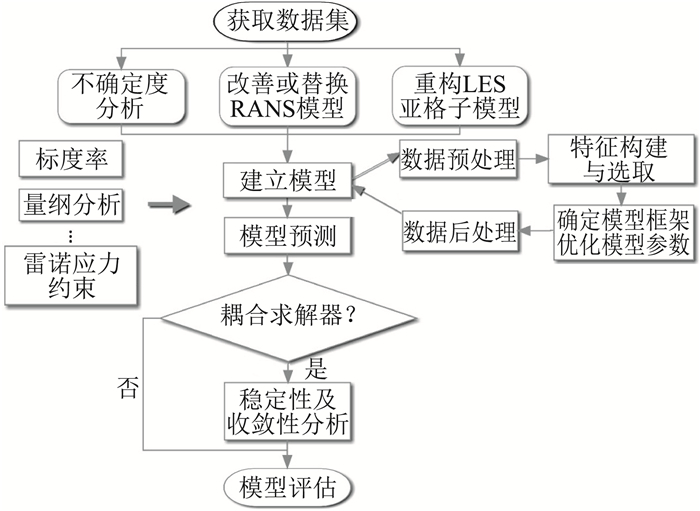图 1 机器学习应用于湍流研究的主要研究方向及流程 Fig.1 Main research direction and process of machine learning applied to turbulence study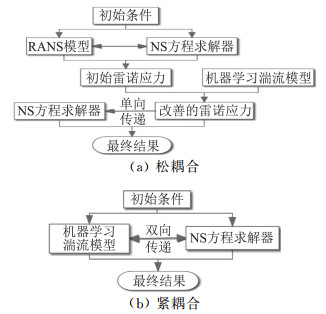图 2 机器学习湍流模型与CFD求解器的耦合 Fig.2 Coupling of machine learning turbulence model with CFD solver
1 机器学习建模过程

1.1 特征选择

1.2 输入输出数据处理

 $\varPi=\left\{\begin{array}{ll}{\tilde{\varPi}, } & {\text { if } \qquad\left(\nabla^{2} \overline{\omega}\right)(\widetilde{\varPi})>0} \\ {0, } & {\text { otherwise }}\end{array}\right.$ (1)

Ling等人对模型预测的各向异性值进行如下约束，

 $\left\{\begin{array}{l}{-\frac{1}{3} \leq b_{i i} \leqslant \frac{2}{3}, \quad \xi_{1} \geqslant\left(3\left|\xi_{2}\right|-\xi_{2}\right) / 2} \\ {-\frac{1}{2} \leqslant b_{i j} \leqslant \frac{1}{2}, \quad \text { for } j \neq i, \quad \xi_{1} \leqslant \frac{1}{3}-\xi_{2}}\end{array}\right.$ (2)
1.3 模型框架2 机器学习在湍流模型中的应用

2.1 湍流模型的不确定度分析

2.2 改善湍流模型

RANS模型由于存在结构和参数上的不确定度，计算精度及适用范围会受到不同程度的限制。对于某些存在激波-边界层干扰的流动或分离流，RANS模型的计算结果往往存在一定的偏差甚至不准确，因此，研究者希望根据高分辨率数据来减小RANS模型计算的偏差，或者使之能够用于分离流的计算。这种采用机器学习方法来改善RANS模型的研究思路大致可分为两类：一种是通过改变模型的控制方程形式，如乘以修正系数或给方程增加源项；另一种是在RANS模型基础上构造偏差函数，然后将RANS模型和偏差函数的计算结果叠加，作为最终的雷诺应力值。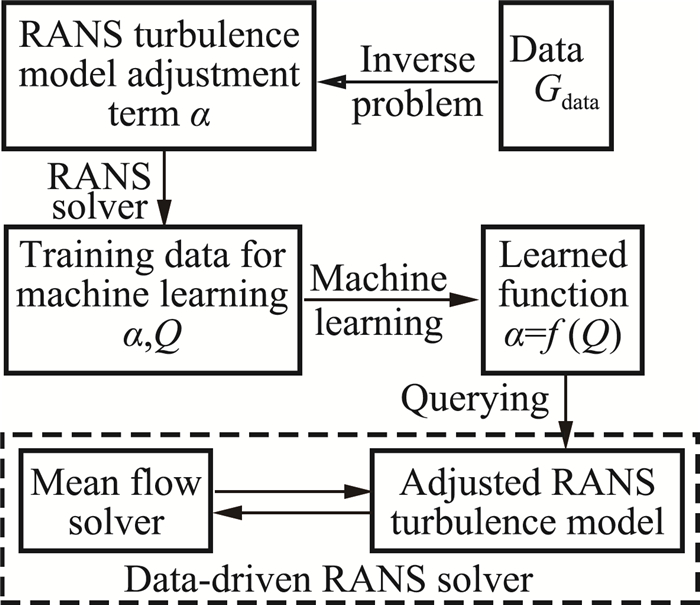图 3 数据驱动的RANS求解器的构建过程 Fig.3 Process for building a data-driven RANS solver
 $\frac{D \widetilde{v}}{D t}=P(\widetilde{v}, U)-D(\widetilde{v}, U)+T(\widetilde{v}, U)$ (3)

 $\frac{D \widetilde{v}}{D t}=\beta(x) P(\widetilde{v}, U)-D(\widetilde{v}, U)+T(\widetilde{v}, U)$ (4)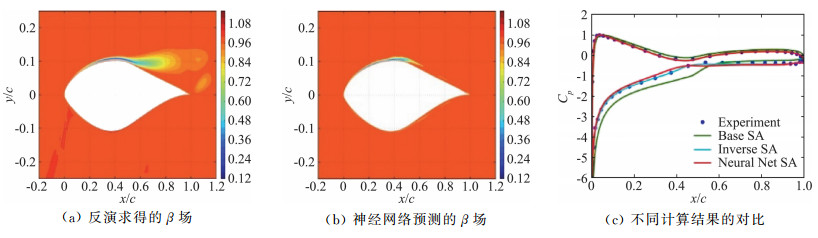图 4 S809翼型在α=14°和Re=2×106时的模型预测结果对比 Fig.4 Comparison of inverse and NN-augmented predictions (using data-set P) for S809 airfoil at α =14°and Re=2×106

 $\tau_{i j}=\tau_{i j, R A N S}+\Delta \tau_{i j, \text { Model }}$ (5)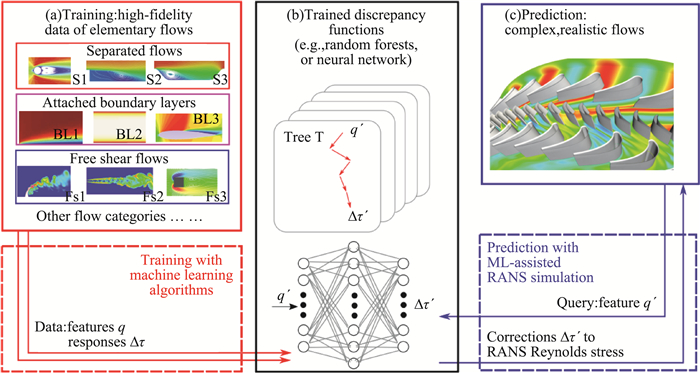图 5 湍流预测建模的基于物理信息的机器学习(PIML)框架 Fig.5 Proposed physics-informed machine learning (PIML) framework for predictive turbulence modeling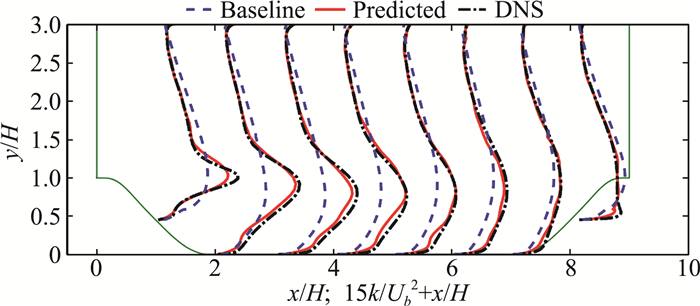图 6 湍流基模型, DNS和PIML预测的湍动能的轮廓对比图(用周期山作训练数据) Fig.6 Profiles of turbulence kinetic energy corresponding to baseline, PIML-predicted and DNS results (flows over periodic hills as training database)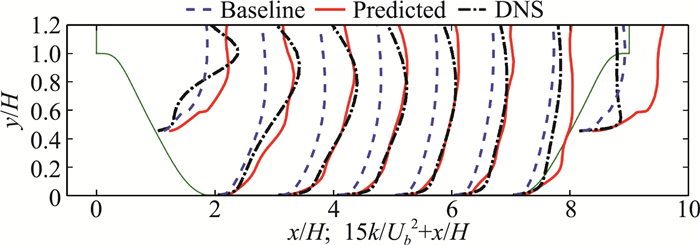图 7 湍流基模型, DNS和PIML预测的湍动能的轮廓对比图(用波形管道和弯曲后向台阶作训练数据) Fig.7 Profiles of turbulence kinetic energy corresponding to baseline, PIML-predicted and DNS results (flows over wavy channels and curved backward facing step as training database)

2.3 替代湍流模型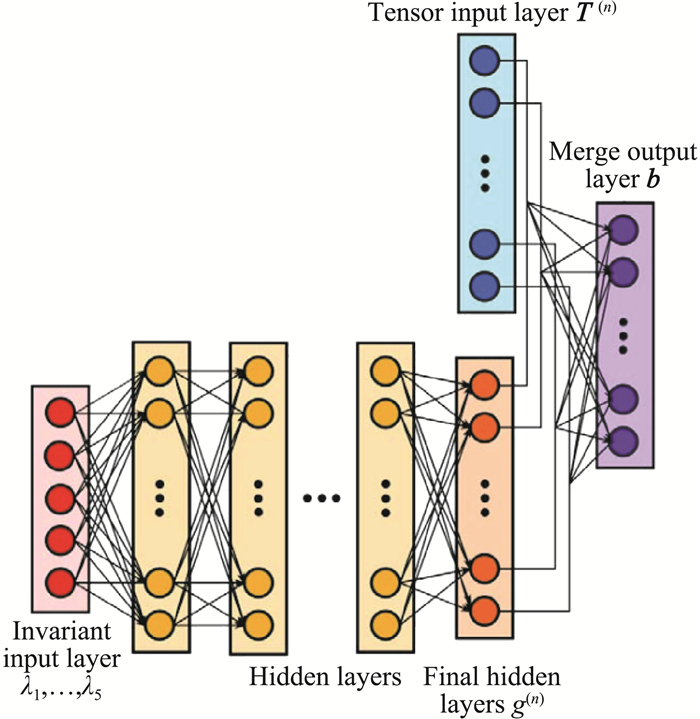图 8 张量基神经网络架构示意图 Fig.8 Schematic of tensor basis neural network architectures
 $\boldsymbol{b}=\sum\limits_{n=1}^{10} g^{(n)}\left(\lambda_{1}, \ldots, \lambda_{5}\right) \boldsymbol{T}^{(n)}$ (6)

 $\boldsymbol{T}^{(1)}=\boldsymbol{S}\\ \boldsymbol{T}^{(2)}=\boldsymbol{S} \boldsymbol{R}-\boldsymbol{R} \boldsymbol{S}\\ \boldsymbol{T}^{(3)}=\boldsymbol{S}^{2}-\frac{1}{3} \boldsymbol{I} \cdot {Tr}\left(\boldsymbol{S}^{2}\right)\\ \boldsymbol{T}^{(4)}=\boldsymbol{R}^{2}-\frac{1}{3} \boldsymbol{I} \cdot {Tr}\left(\boldsymbol{R}^{2}\right)\\ \boldsymbol{T}^{(5)}=\boldsymbol{R} \boldsymbol{S}^{2}-\boldsymbol{S}^{2} \boldsymbol{R}\\\boldsymbol{T}^{(6)}=\boldsymbol{R}^{2} \boldsymbol{S}+\boldsymbol{S} \boldsymbol{R}^{2}-\frac{2}{3} \boldsymbol{I} \cdot {Tr}\left(\boldsymbol{S} \boldsymbol{R}^{2}\right)\\ \boldsymbol{T}^{(7)}=\boldsymbol{R} \boldsymbol{S} \boldsymbol{R}^{2}-\boldsymbol{R}^{2} \boldsymbol{S} \boldsymbol{R}\\\boldsymbol{T}^{(8)}=\boldsymbol{S} \boldsymbol{R} \boldsymbol{S}^{2}-\boldsymbol{S}^{2} \boldsymbol{R} \boldsymbol{S}\\ \boldsymbol{T}^{(9)}=\boldsymbol{R}^{2} \boldsymbol{S}^{2}+\boldsymbol{S}^{2} \boldsymbol{R}^{2}-\frac{2}{3} \boldsymbol{I} \cdot {Tr}\left(\boldsymbol{S}^{2} \boldsymbol{R}^{2}\right)\\\boldsymbol{T}^{(10)}=\boldsymbol{R} \boldsymbol{S}^{2} \boldsymbol{R}^{2}-\boldsymbol{R}^{2} \boldsymbol{S}^{2} \boldsymbol{R}$ (7)
 $\begin{array}{l}{\lambda_{1}={Tr}\left(\boldsymbol{S}^{2}\right), \lambda_{2}={Tr}\left(\boldsymbol{R}^{2}\right), \lambda_{3}={Tr}\left(\boldsymbol{S}^{3}\right)}, \\ {\lambda_{4}={Tr}\left(\boldsymbol{R}^{2} \boldsymbol{S}\right), \lambda_{5}={Tr}\left(\boldsymbol{R}^{2} \boldsymbol{S}^{2}\right)}\end{array}$ (8)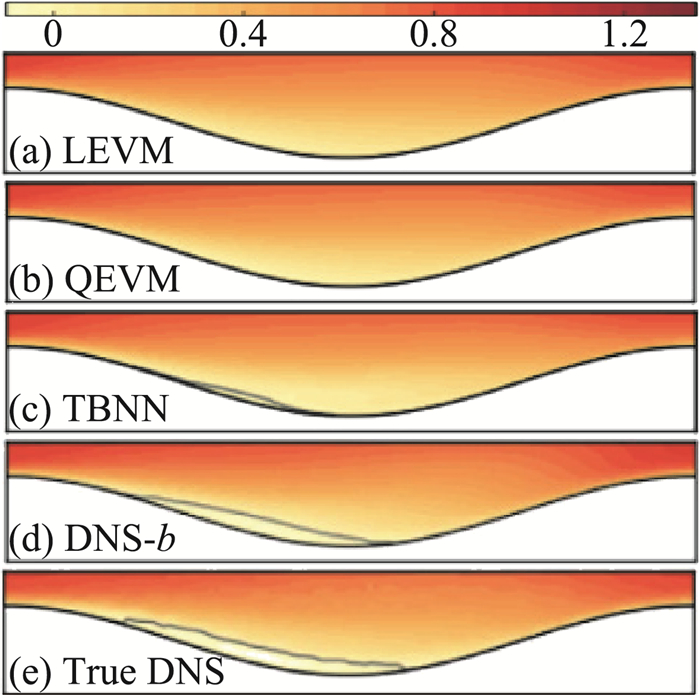图 9 波形壁测试算例中的流向速度云图(分离区用灰线描绘) Fig.9 Contours of streamwise velocity normalized by bulk velocity in the wavy wall test case, zoomed into the near-wall region, (separated regions outlined in grey)

Zhu等人探讨了直接构建纯数据驱动的湍流黑箱代数模型, 并实现了与求解器之间的耦合计算(图 10)。研究结果表明，基于NACA0012翼型的三个亚声速状态算例，所构建的模型可以实现与SA模型相当的精度和更高的计算效率，并对计算状态(图 11)和几何外形(图 12)具备一定的泛化能力，验证了替代模型的可行性。与修正模型不同，替代模型的性能对最终摩擦阻力的计算结果起决定作用，而且对求解器的收敛性也有重要影响。换言之，模型构建过程对模型输出的精度和光滑性以及模型的稳定性都有很高要求。对于回归问题，损失函数决定了量级大的值比量级小的值容易取得更高的拟合精度，然而，边界层内(尤其是高雷诺数时)的剧烈变化容易导致湍流变量的明显的尺度效应。因此，替代模型很难在整个的训练数据空间中都保证较高的精度。模型性能欠佳的“边缘区域”容易出现异值，继而影响模型输出的光滑性。此外，将所构建的模型嵌入求解器后，模型预测在迭代过程中会存在一定程度的不稳定，残差在下降过程中出现震荡，如图 13所示。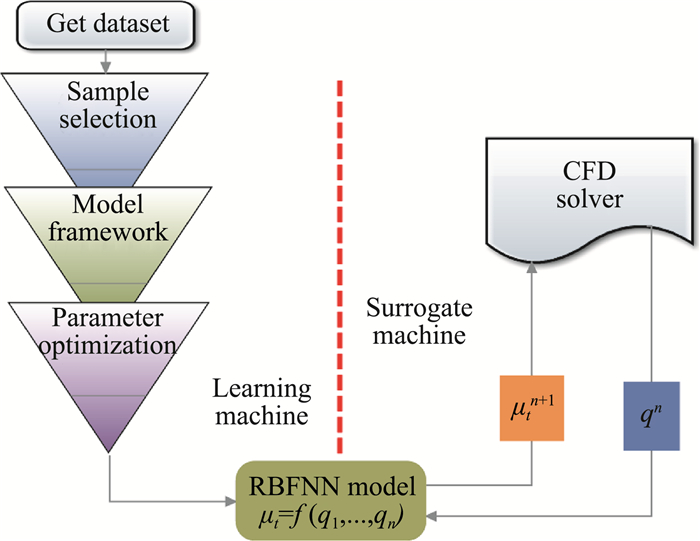图 10 构建学习器和代理器的流程图(q和μt分别表示输入特征和涡黏) Fig.10 Flow chart for building the learning machine and surrogate machine, (q and μt mean the input features and eddy viscosity, respectively)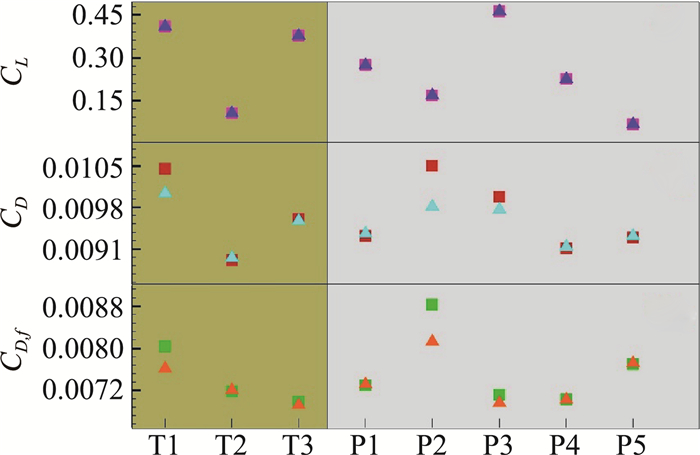图 11 训练和预测算例中SA (方框)和RBFNN (三角形)计算的力系数结果对比 Fig.11 Comparison of the force coefficient calculated by SA (square) and RBFNN (delta) for both training and predicting cases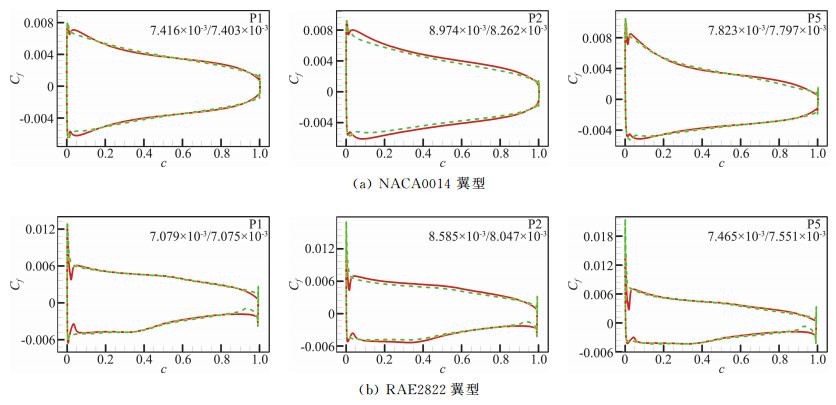图 12 预测算例P1、P2和P5的摩擦阻力系数分布对比(图中数据表示SA/RBFNN计算的摩擦阻力系数CD, f) Fig.12 Predictions for NACA0014 and RAE2822 airfoil at P1, P2 and P5 cases. (the data inside are CD, f values calculated by SA/RBFNN model)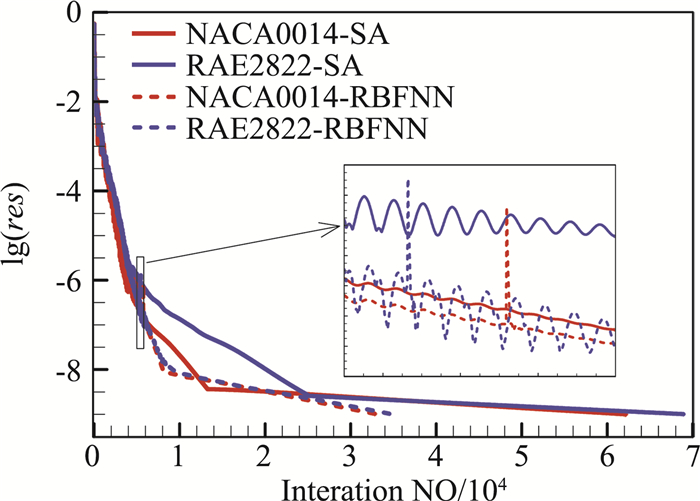图 13 P5算例的残差下降过程曲线图 Fig.13 Residual evolution at P5 case

 $\frac{\partial \overline{\omega}}{\partial t}+J(\overline{\omega}, \overline{\varphi})=\frac{1}{R e} \nabla^{2} \overline{\omega}+\varPi$ (9)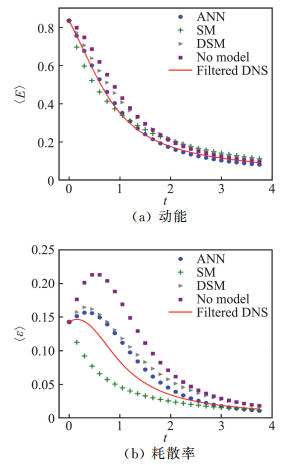图 14 不同计算方法之间可分辨的动能和耗散率的时间演化图 Fig.14 Temporal evolution of resolved kinetic energy and dissipation by different simulations
2.4 模型的鲁棒性与稳定性

3 总结与展望

1) 湍流建模输入特征的提取与优化。单纯地依靠海量数据作为直接输入，通过简单增加神经网络模型的深度和宽度来构建复杂映射关系并不是一种好的建模策略。如何对输入数据进行特征提取，特别是结合前人在湍流理论中已经取得的物理规律和经验，如选择不变量、利用各种标度率、量纲分析方法等，精简输入信息、简化网络模型的维度和复杂性，从而实现模型的紧凑性和泛化能力的提升。当然，也可以借鉴人工智能方法开展输入信息的特征构建与提取，如Featuretools等。

2) 湍流模型与N-S方程耦合求解过程中的稳定性与收敛性。随着机器学习在湍流模型化中的作用越来越明显，模型的输出结果对速度场的影响也越大。一方面，机器学习方法所构建的模型本身就有可能出现局部峰值和光滑性差的输出值，降低计算精度。另一方面，模型训练大多是基于某些时刻或流场中某些位置的数据进行的，模型难以包含流场在迭代过程中的全部信息。当模型参与求解器的每一步迭代时，模型的扰动会导致N-S方程求解的流场扰动，继而反馈为模型的输入偏差。这种反馈会造成迭代过程的发散，即使对于单向耦合策略也有可能造成求解过程的失稳。如果模型的稳定性较差，特别是在非线性很强的高雷诺数及大分离等复杂流动中，模型的误差随着迭代过程的累积容易在速度场中被放大，导致N-S方程求解器的最终计算结果变差。特别是采用高维深度学习网络，所带来的局部极值对耦合过程的稳定性和收敛性都带来了不利影响。

3) N-S方程和湍流模型耦合求解过程中的多平衡态问题。基于收敛状态的计算结果构建的模型在耦合求解器后有时还会出现多态解的问题。由于N-S方程和湍流的非线性和复杂性，模型的计算结果也会受初始条件等的影响，将训练后的模型嵌入N-S求解器后，最终的计算结果有可能会收敛为其它平衡态的解。关于模型与求解器的耦合问题值得进一步研究。

  BATCHELOR G, PROUDMAN I. The effect of rapid distortion of a fluid in turbulent motion[J]. The Quarterly Journal of Mechanics and Applied Mathematics, 1954, 7(1): 83-103. DOI:10.1093/qjmam/7.1.83  HUNT J C, CARRUTHERS D J. Rapid distortion theory and the 'problems' of turbulence[J]. Journalof Fluid Mechanics, 1990, 212: 497-532. DOI:10.1017/S0022112090002075  KLINE S J, REYNOLDS W C, SCHRAUB F, et al. The structure of turbulent boundary layers[J]. Journal of Fluid Mechanics, 1967, 30(4): 741-773. DOI:10.1017/S0022112067001740  CHOU P Y. On velocity correlations and the solutions of the equationsof turbulent fluctuation[J]. Quarterly of Applied Mathematics, 1945, 3(1): 38-54. DOI:10.1090/qam/1945-03-01  佘振苏, 唐帆, 肖梦娟. 面向精准工程湍流模型的理论研究[J]. 空气动力学学报, 2019, 37(1): 1-18. SHE Z S, TANG F, XIAO M J. Structural ensemble dynamics theory for engineering turbulenc models[J]. Acta Aerodynamica Sinica, 2019, 37(1): 1-18. DOI:10.7638/kqdlxxb-2018.0249 (in Chinese)  CHEN S, XIA Z, PEI S, et al. Reynolds-stress-constrained large-eddy simulation of wall-bounded turbulent flows[J]. Journal of Fluid Mechanics, 2012, 703: 1-28. DOI:10.1017/jfm.2012.150  HE G, JIN G, YANG Y. Space-time correlations and dynamiccoupling in turbulent flows[J]. Annual Review of Fluid Mechanics, 2017, 49: 51-70. DOI:10.1146/annurev-fluid-010816-060309  周恒, 张涵信. 号称经典物理留下的世纪难题"湍流问题"的实质是什么?[J]. 中国科学:物理学力学天文学, 2012, 42(1): 1-5. ZHOU H, ZHANG H X. What is the essence of the so-called century lasting difficult problem in classic physics, the "problem of turbulence "?[J]. Sci Sin-Phys Mech Astron, 2012, 42(1): 1-5. (in Chinese)  SLOTNICK J, KHODADOUST A, ALONSO J, et al. CFD vision 2030 study: A path to revolutionary computational aerosciences[R]. NASA CR-2014-218178. https://ntrs.nasa.gov/archive/nasa/casi.ntrs.nasa.gov/20140003093.pdf  DURBIN P A. Some recent developments in turbulence closure modeling[J]. Annual Review of Fluid Mechanics, 2015, 50(1): 77-103.  MOLLICONE J P, BATTISTA F, GUALTIERI P, et al. Effect of geometry and Reynolds number on the turbulent separated flow behind a bulgein a channel[J]. Physics, 2017, 781(7): 100-133.  BALDWIN B, LOMAX H. Thin-layer approximation and algebraic model for separated turbulentflows[C]//16th Aerospace Sciences Meeting, Huntsville, 1978: 257. https://arc.aiaa.org/doi/abs/10.2514/6.1978-257  LAUNDER B E, SPALDING D B. The numerical computation of turbulent flows[J]. Computer Methods in Applied Mechanics & Engineering, 1974, 103(2): 269-289.  WILCOX D C. Reassessment of the scale-determining equation for advanced turbulence models[J]. AIAA Journal, 1988, 26(11): 1299-1310. DOI:10.2514/3.10041  SPALARTP, ALLMARAS S. A one-equation turbulence model for aerodynamic flows[C]//30th Aerospace Sciences Meeting and Exhibit, 1992: 439. https://www.researchgate.net/publication/236888804_A_One-Equation_Turbulence_Model_for_Aerodynamic_Flows  MENTER F R. Two-equation eddy-viscosity turbulence models for engineering applications[J]. AIAA Journal, 1994, 32(8): 1598-1605. DOI:10.2514/3.12149  SAFFMAN P G. A model for inhomogeneous turbulent flow[J]. Proc R Soc Lond A, 1970, 317(1530): 417-433. DOI:10.1098/rspa.1970.0125  SPEZIALE C G, ABID R, ANDERSON E C. Critical evaluation of two-equation models for near-wall turbulence[J]. AIAA Journal, 1992, 30(2): 324-331. DOI:10.2514/3.10922  LAUNDER B E, SHARMA B. Application of the energy-dissipation model of turbulence to the calculation of flow near a spinning disc[J]. Letters in Heat and Mass Transfer, 1974, 1(2): 131-137. DOI:10.1016/0094-4548(74)90150-7  LAUNDER B E, REECE G J, RODI W. Progress in the development of a Reynolds-stress turbulence closure[J]. Journal of Fluid Mechanics, 1975, 68(3): 537-566. DOI:10.1017/S0022112075001814  CHEN X, HUSSAIN F, SHE Z S. Quantifying wall turbulence via a symmetry approach. Part 2. Reynolds stresses[J]. J Fluid Mech, 2018, 850: 401-438. DOI:10.1017/jfm.2018.405  SHE Z S, CHEN X, HUSSAIN F. Quantifying wall turbulence via a symmetry approach:a Lie group theory[J]. Journal of Fluid Mechanics, 2017, 827: 322-356. DOI:10.1017/jfm.2017.464  ZHANG Y, CHAN W, JAITLY N. Very deep convolutional networks for end-to-end speech recognition[C]//2017 IEEE International Conference on Acoustics, Speech and Signal Processing (ICASSP), 2017: 4845-4849. https://arxiv.org/abs/1610.03022  LIN T Y, ROYCHOWDHURY A, MAJI S. Bilinear convolutional neural networks for fine-grained visual recognition[J]. IEEE Transactions on PatternAnalysis & Machine Intelligence, 2018, 40(6): 1309-1322.  WEN X, LIU Y, LI Z, et al. Data mining of a clean signal from highly noisy data based on compressed data fusion:A fast-responding pressure-sensitive paint application[J]. Physics of Fluids, 2018, 30(9): 097103. DOI:10.1063/1.5046681  KOU J, ZHANG W. A hybrid reduced-order framework for complex aeroelastic simulations[J]. Aerospace Science and Technology, 2019, 84: 880-894. DOI:10.1016/j.ast.2018.11.014  MILANO M, KOUMOUTSAKOS P. Neural network modeling for near wall turbulent flow[J]. Journal of Computational Physics, 2002, 182(1): 1-26.  HOCEVAR M, SIROK B, GRABEC I. A Turbulent-wake estimation using radial basis function neural networks[J]. Flow Turbulence & Combustion, 2005, 74(3): 291-308.  DURAISAMY K, SPALART P R, RUMSEY C L. Status, emerging ideas and future directions of turbulence modeling research in aeronautics[R]. NASA TM-2017-219682. https://ntrs.nasa.gov/archive/nasa/casi.ntrs.nasa.gov/20170011477.pdf  TRACEY B D, DURAISAMY K, ALONSO J J. A machine learning strategy to assist turbulence model development[C]//53rd AIAA Aerospace Sciences Meeting, 2015: 1287. https://deepblue.lib.umich.edu/bitstream/handle/2027.42/140498/6.2015-1287.pdf?sequence=1&isAllowed=y  ZHANG Z J, DURAISAMY K. Machine learning methods for data-driven turbulence modeling[C]//22nd AIAA Computational Fluid Dynamics Conference, 2015: 2460. https://arc.aiaa.org/doi/abs/10.2514/6.2015-2460  DURAISAMY K, ZHANG Z J, SINGH A P. New approaches in turbulence and transition modeling using data-driven techniques[C]//53rd AIAA Aerospace Sciences Meeting, 2015: 1284. https://deepblue.lib.umich.edu/bitstream/handle/2027.42/140497/6.2015-1284.pdf?sequence=1&isAllowed=y  SINGH A P, DURAISAMY K, ZHANG Z J. Augmentation of turbulence models using field inversion and machine learning[C]//55th AIAA Aerospace Sciences Meeting, 2017: 0993.  WANG J X, WU J L, XIAO H. A physics informed machine learning approach for reconstructing Reynolds stress modeling discrepancies based on DNS data[J]. Phys Rev Fluids, 2017(3): 1-22.  LING J, KURZAWSKI A, TEMPLETON J. Reynolds averaged turbulence modelling using deep neural networks with embedded invariance[J]. Journal of Fluid Mechanics, 2016, 807: 155-166. DOI:10.1017/jfm.2016.615  GAMAHARA M, HATTORI Y. Searching for turbulence models by artificial neural network[J]. Physical Review Fluids, 2017, 2(5): 054604. DOI:10.1103/PhysRevFluids.2.054604  MAULIK R, SAN O, RASHEED A, et al. Subgrid modelling for two-dimensional turbulence using neural networks[J]. Journal of Fluid Mechanics, 2019, 858: 122-144. DOI:10.1017/jfm.2018.770  WANG Z, LUO K, LI D, et al. Investigations of data-driven closure for subgrid-scale stress in large-eddy simulation[J]. Physics of Fluids, 2018, 30(12): 125101. DOI:10.1063/1.5054835  NATHAN K J. Deep learning in fluid dynamics[J]. Journal of Fluid Mechanics, 2017, 814: 1-4. DOI:10.1017/jfm.2016.803  LING J, TEMPLETON J. Evaluation of machine learning algorithms for prediction of regions of high Reynolds averaged Navier-Stokes uncertainty[J]. Physics of Fluids, 2015, 27(8): 042032-042094.  ZHANG Z, SONG X D, YE S R, et al. Application of deep learning method to Reynolds stress models of channel flow based on reduced-order modeling of DNS data[J]. Journal of Hydrodynamics, 2019, 31(1): 58-65. DOI:10.1007/s42241-018-0156-9  GUYON I, ELISSEEFF A. An introduction to feature extraction[M]. Feature extraction, Springer, Heidelberg, 2006: 1-25.  LING J, JONES R, TEMPLETON J. Machine learning strategies for systems with invariance properties[J]. Journal of Computational Physics, 2016, 318: 22-35. DOI:10.1016/j.jcp.2016.05.003  SINGH A P, MEDIDA S, DURAISAMY K. Machine-learning-augmented predictive modeling of turbulent separated flows over airfoils[J]. AIAA Journal, 2017, 2215-2227.  BECK A D, FLAD D G, MUNZ C D. Deep neural networks for data-driven turbulence models[J]. Research Gate Preprint, 2018.  ZHU L, ZHANG W, KOU J, et al. Machine learning methods for turbulence modeling in subsonic flows around airfoils[J]. Physics of Fluids, 2019, 31(1): 015105. DOI:10.1063/1.5061693  EDELING W, CINNELLA P, DWIGHT R P, et al. Bayesian estimates of parameter variability in the k-ε turbulence model[J]. Journal of Computational Physics, 2014, 258: 73-94. DOI:10.1016/j.jcp.2013.10.027  CHEUNG S H, OLIVER T A, PRUDENCIO E E, et al. Bayesian uncertainty analysis with applications to turbulence modeling[J]. Reliability Engineering & System Safety, 2011, 96(9): 1137-1149.  OLIVER T A, MOSER R D. Bayesian uncertainty quantification applied to RANS turbulence models[J]. Journal of Physics:Conference Series, 2011, 042032.  MARGHERI L, MELDI M, SALVETTI M, et al. Epistemic uncertainties in RANS model free coefficients[J]. Computers & Fluids, 2014, 102: 315-335.  XIAO H, WANG J X, GHANEM R G. A random matrix approach for quantifying model-form uncertainties in turbulence modeling[J]. Computer Methods in Applied Mechanics & Engineering, 2017, 313: 941-965.  DOW E, WANG Q. Quantification ofstructural uncertainties in the k-w turbulence model[C]//52nd AIAA/ASME/ASCE/AHS/ASC Structures, Structural Dynamics and Materials Conference. 19th AIAA/ASME/AHS Adaptive Structures Conference, 2011: 1762. https://www.researchgate.net/publication/267701492_Quantification_of_Structural_Uncertainties_in_the_k_-w_Turbulence_Model  PLATTEEUW P, LOEVEN G, BIJL H. Uncertainty quantification applied to the k-epsilon model of turbulence using the probabilistic collocation method[C]//49th AIAA/ASME/ASCE/AHS/ASC Structures, Structural Dynamics, and Materials Conference. 16th AIAA/ASME/AHS Adaptive Structures Conference, 2008: 2150. https://www.researchgate.net/publication/268478177_Uncertainty_Quantification_Applied_to_the_k-epsilon_Model_of_Turbulence_Using_the_Probabilistic_Collocation_Method  YARLANKI S, RAJENDRAN B, HAMANN H. Estimation of turbulence closure coefficients for data centers using machine learning algorithms[C]//Thermal and Thermomechanical Phenomena in Electronic Systems, 2012: 38-42. https://www.researchgate.net/publication/261391669_Estimation_of_turbulence_closure_coefficients_for_data_centers_using_machine_learning_algorithms  LING J, KURZAWSKI A. Data-driven adaptive physics modeling for turbulence simulations[C]//23rd AIAA Computational Fluid Dynamics Conference, 2017: 3627. https://arc.aiaa.org/doi/abs/10.2514/6.2017-3627  LING J. Using machine learning to understand and mitigate model form uncertainty in turbulence models[C]//IEEE International Conference on Machine Learning and Applications, 2015: 813-818. https://www.researchgate.net/publication/300409020_Using_Machine_Learning_to_Understand_and_Mitigate_Model_Form_Uncertainty_in_Turbulence_Models  SINGH A P, DURAISAMY K. Using field inversion to quantify functional errors in turbulence closures[J]. Physics of Fluids, 2016, 28(4): 045110. DOI:10.1063/1.4947045  XIAO H, CINNELLA P. Quantification of model uncertainty in RANS simulations:A review[J]. Progress in Aerospace Sciences, 2019, 108: 1-31. DOI:10.1016/j.paerosci.2018.10.001  XIAO H, WU J, WANG J, et al. Physics informed machine learning for predictive turbulence modeling: Progress and perspectives[R]. AIAA 2017-1712, 2017. https://www.researchgate.net/publication/312267765_A_Physics-Informed_Machine_Learning_Approach_of_Improving_RANS_Predicted_Reynolds_Stresses  WANG J X, HUANG J, DUAN L, et al. Prediction of Reynolds stresses in high-Mach-number turbulent boundary layers using physics-informed machine learning[J]. Theoretical and Computational Fluid Dynamics, 2019, 33(1): 1-19.  POPE S. A more general effective-viscosity hypothesis[J]. Journal of Fluid Mechanics, 1975, 72(2): 331-340. DOI:10.1017/S0022112075003382  SARGHINI F, DE FELICE G, SANTINI S. Neural networks based subgrid scale modeling in large eddy simulations[J]. Computers & Fluids, 2003, 32(1): 97-108.  SU D, ZHANG H, CHEN H, et al. Is robustness the cost of accuracy?-A comprehensive study on the robustness of 18 deep image classification models[C]//Proceedings of the European Conference on Computer Vision (ECCV), 2018: 631-648.  WU J L, XIAO H, PATERSON E G. Physics-informed machine learning approach for augmenting turbulence models:A comprehensive framework[J]. Physical Review Fluids, 2018, 3: 074602. DOI:10.1103/PhysRevFluids.3.074602  WU J L, XIAO H, SUN R, et al. Reynolds averaged Navier-Stokes equations with explicit data-driven Reynolds stress closure can be ill-conditioned[J]. Journal of Fluid Mechanics, 2019, 869: 553-586. DOI:10.1017/jfm.2019.205